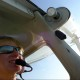Home » News » Density and Pressure AltitudeHot summer flying makes for low density altitude.

# Density and Pressure Altitude

When we go flying, we always calculate pressure and density altitude of our aerodrome and for our cruising altitude. Why do we do this? Recall the definitions of density altitude (DA) and pressure altitude (PA).

PA is the “height above sea level corresponding to a given barometric pressure under standard air conditions” (FGU, page 41).  What are standard air conditions?  Standard air conditions state that at sea level, the air has a pressure of 29.92″ Hg and temperature of 15 degrees C.  As we increase in altitude, the air cools at the adiabatic lapse rate of 1.98 degrees per 1000 feet.  This allows us to calculate our altitude for pressure given the pressure reading at our aerodrome. To calculate this, we always subtract our current altimeter reading from standard pressure of 29,92,  multiply by 1000 then add it to the actual AGL elevation of the aerodrome.

For example, at Springbank, when the altimeter reading is 30.15:

29.92 – 30.15 = -0.23 * 1000 = – 230  then add to the altitude of CYBW which is 3940:  3940 + (-230) = 3710.   This means that the actual pressure, at standard atmospheric conditions, is lower than actual field height.

The reason we need to know pressure altitude is because our altimeter is a pressure altimeter.  It is important to set the altimeter because knowing the actual height of the airplane is of vital importance.  An altimeter setting that is too high will give an altimeter reading that is too high, a low setting will show a reading that is lower than we actually are.   Generally, most standard altimeters doesn’t go above a pressure reading higher than 31.0 0″ Hg. Such high pressure readings are found in very cold, dry air masses.   To correct for this, the pilot can add 100 feet for each 0.10 ” reading above this figure.  This will give the true altitude of the aircraft.

So why do we need to know pressure altitude anyway? When we do flight planning, we need to calculate it for our departing aerodrome not only to have the proper pressure setting for our altimeter, but also because the performance of the aircraft is based on pressure altitude.  These are more direct engine performance things, like fuel burn, true airspeed and engine thrust. We always look at our POH to find the true airspeed which we base on pressure altitude, like climb and cruise performance.Hot, hazy summer day produces high density altitude and reduced performance

Density altitude is based on pressure altitude. It is simply pressure altitude corrected for temperature.   Once we calculate pressure altitude, we use the following formula:

DA = PA + [ 100 * (Actual Temperature – Standard Temperature) ]

Actual temperature is self explanatory, if we are looking for temperature of our aerodrome it will be given in ATIS or if we are looking for the temperature of our cruising altitude that is available in the Upper Wind Forecasts (FD’s).  To get  standard temperature recall the definition of standard air conditions: cools at the adiabatic lapse rate of 1.98 degrees per 1000 feet. So we can calculate this ourselves to know what the standard temperature should be at our chosen altitude.  For instance, for my last flight I flew at 6000 feet and the standard temperature at this altitude is (6 * 2 = )12 degrees less than 15 degrees, or 3 degrees.  We can also refer to our aircraft’s POH, where standard temperature for the pressure altitude is listed.

Density altitude is important to know for lift and aerodynamics.  Have you ever taken off from an airport on a hot, hazy summer day and noticed the decrease in engine performance?  On these days it takes longer to accelerate and become airborne because the air density is behaving as if it were in much higher altitude – where there is less air for the aircraft to “grab” onto.  DA is important for calculating safe fuel and payload permissible for takeoff.

High density altitude makes take off and climb take longer. Last summer, on 30 degree days when flying circuits I noticed that I was consistently not reaching circuit altitude until I was well into my downwind leg. Typically, I reach the altitude on crosswind.

What gives good performance?  Low altitude (higher air density), cold and dry. Bad performance? High altitude (lower air density), hot and humid.

Also read how altitude affects performance.1.